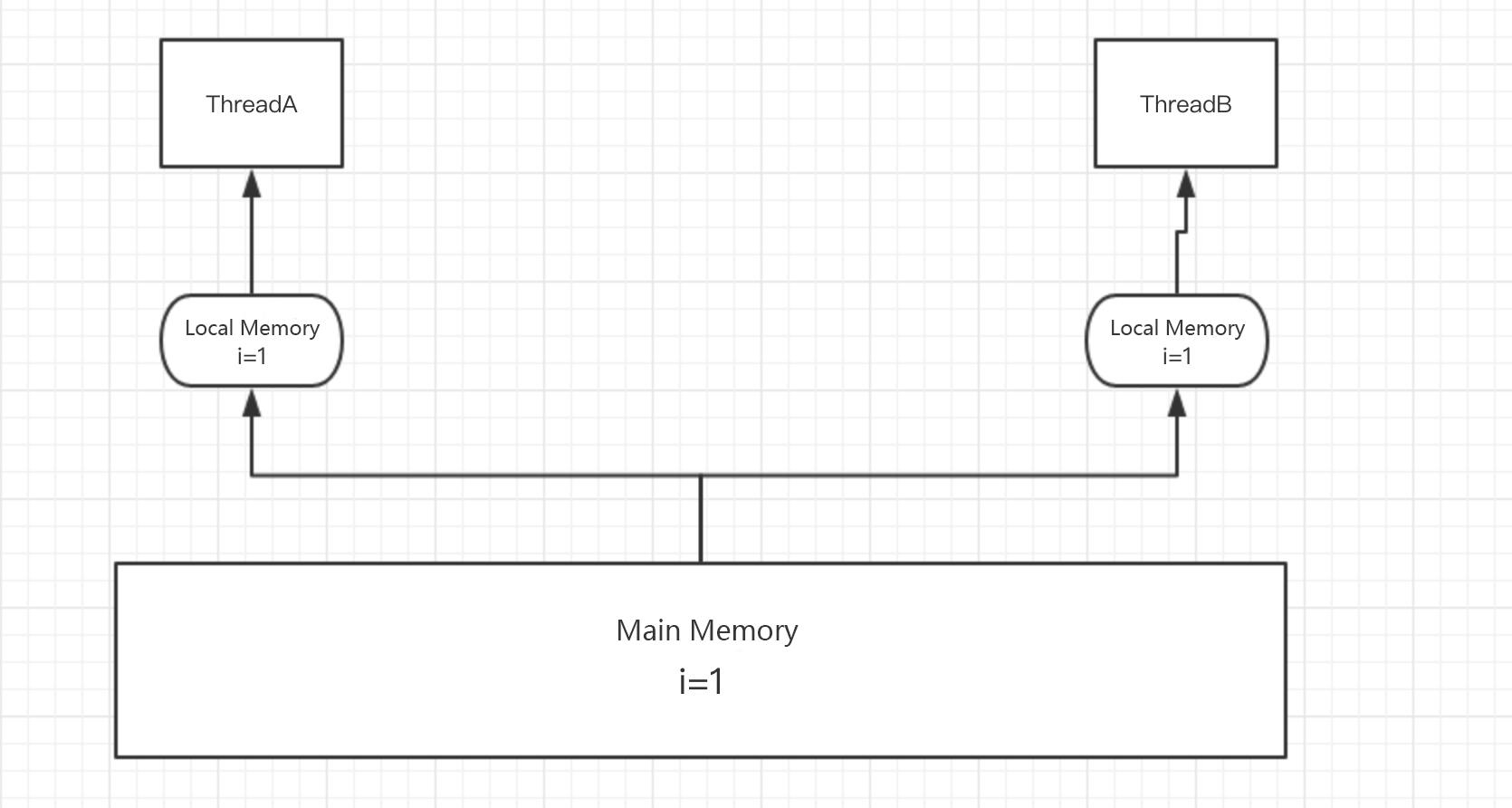# Application of Volatile

## Memory Visibility

Since the Java Memory Model (JMM) states that all variables are stored in main memory, and each thread has its own working memory (cache).

When a thread is working, it needs to copy the data from the main memory to the working memory. This way, any operation on the data is based on the working memory (which is more efficient) and cannot directly manipulate the data in the main memory or the working memory of other threads, and then flush the updated data to the main memory afterwards.

The main memory mentioned here can be simply thought of as heap memory, while the working memory can be thought of as stack memory.

As shown in the figure below.So during concurrent operation it may happen that the data read by thread B is the data before the update by thread A.

Obviously, this is a problem, and volatile can solve the problem.

When a variable is modified with volatile, writes to it by any thread are immediately flushed to main memory and force the thread that cached the variable to clear the data and have to read the latest data from main memory again.

The volatile modification does not allow threads to fetch data directly from main memory; they still need to copy the variable into working memory.

### Application of Memory Visibility

When we need to communicate between two threads based on main memory, the variable to be communicated with must be modified with volatile.

``````public class Volatile implements Runnable{
private static volatile boolean flag = true ;
@Override
public void run() {
while (flag){
}
}
public static void main(String[] args) throws InterruptedException {
Volatile aVolatile = new Volatile();
Scanner sc = new Scanner(System.in);
while(sc.hasNext()){
String value = sc.next();
if(value.equals("1")){
@Override
public void run() {
}
}).start();
break ;
}
}
System.out.println("The main thread has exited!") ;
}
flag = false ;
}
}
``````

The main thread has modified the flag bit to make thread A stop immediately, which could be delayed if not modified with volatile.

However, there is a misconception here that such use tends to give the impression that Concurrent operations on volatile-modified variables are thread-safe.

A few words of clarification here: `volatile` doesn’t guarantee thread safety!

The following program:

``````public class VolatileInc implements Runnable{
private static volatile int count = 0 ; //using volatile to modify basic data memory does not guarantee atomicity
@Override
public void run() {
for (int i=0;i<10000 ;i++){
count ++ ;
}
}
public static void main(String[] args) throws InterruptedException {
VolatileInc volatileInc = new VolatileInc() ;
t1.start();
t2.start();
for (int i=0;i<10000 ;i++){
count ++ ;
}
System.out.println("finalCount="+count);
}
}
``````

When we have three threads (t1,t2,main) accumulating an int at the same time, we find that the final value is less than 30000.

This is because although volatile ensures memory visibility and each thread gets the latest value, the count ++ operation is not atomic, and the operations involved in getting the value, self-incrementing, and assigning the value cannot be done at the same time.

You can also use synchronize or lock to ensure atomicity.

You can also replace int with AtomicInteger from the Atomic package, which uses the CAS algorithm to guarantee atomicity.

## Instruction rearrangement

Memory visibility is only one of the semantics of volatile, it also prevents the JVM from performing instruction rearrangement optimizations.

Take a pseudo-code:

``````int a=10 ; //1
int b=20 ; //2
int c= a+b ; //3
``````

A particularly simple piece of code, which ideally would be executed in the order 1>2>3, may be optimized by the JVM to execute in the order 2>1>3.

You can see that no matter how the JVM is optimized, the premise is to ensure that the final result remains the same in a single thread.

If you can’t see the problem here, look at the next piece of pseudo-code:

``````
private static Map<String, String> value;
private static volatile boolean flag = fasle;

// The following method occurs in thread A Initialize Map
public void initMap() {
// time consuming operation
value = getMapValue();//1
flag = true;//2
}

// occur in thread B wait until the Map initialization success for other operations
public void doSomeThing() {
while (!flag) {
sleep();
}
//dosomething
doSomeThing(value);
}
``````

The problem is that when the flag is not modified by volatile, the JVM reorders 1 and 2, resulting in the value being used by thread B before it is even initialized.

So adding volatile prevents this kind of reordering optimization and ensures correctness of business.

### Application of instruction reordering

``````public class Singleton {
private static volatile Singleton singleton;
private Singleton() {
}
public static Singleton getInstance() {
if (singleton == null) {
synchronized (Singleton.class) {
if (singleton == null) {
//prevent instruction reordering
singleton = new Singleton();
}
}
}
return singleton;
}
}
``````

The volatile keyword here is mainly to prevent instruction reordering.

If you don’t use , singleton = new Singleton();, this code is actually divided into three steps.

• Allocate memory space.
• Initialize the object.
• Point the singleton object to the allocated memory address.

Adding volatile is to let the above three steps in order to execute the operation, otherwise it is possible that the second step is executed before the third step, it is possible that a thread gets the singleton object is not yet initialized, so it reports an error.

## Summary

volatile is used a lot in Java concurrency, such as the value in the Atomic package, and the state in the AbstractQueuedLongSynchronizer are defined as volatile to ensure memory visibility.